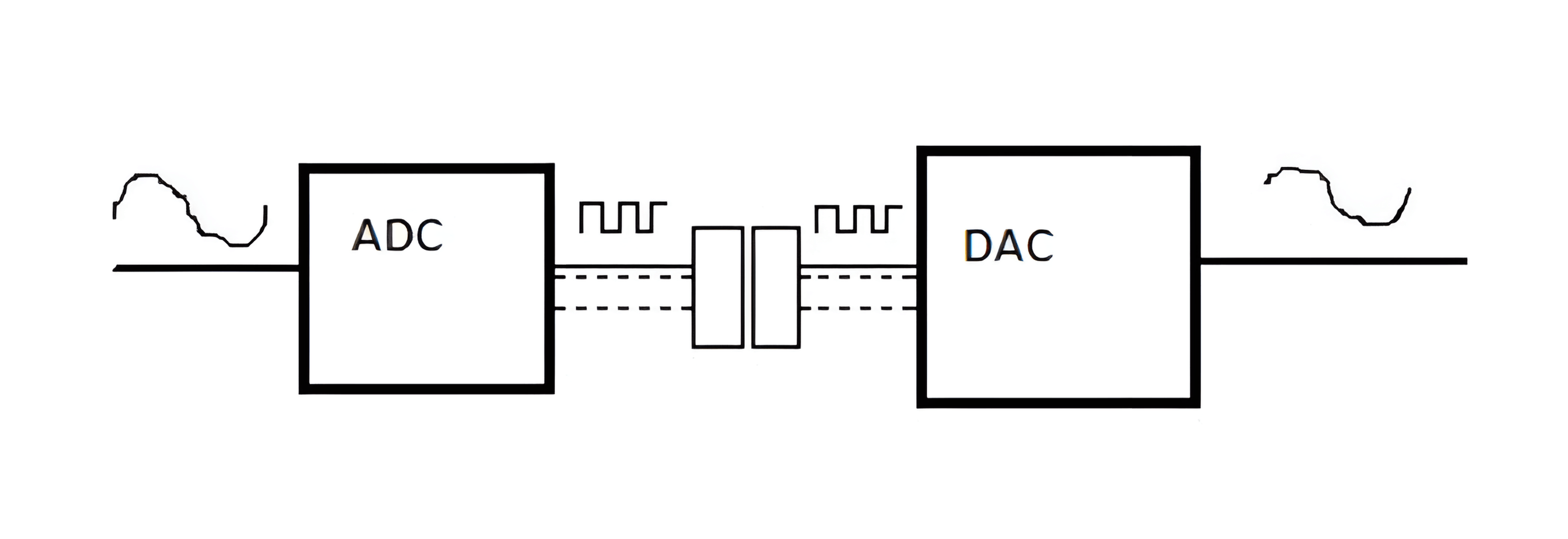### 采样

$f_s≥2\cdot f_{i(max)}$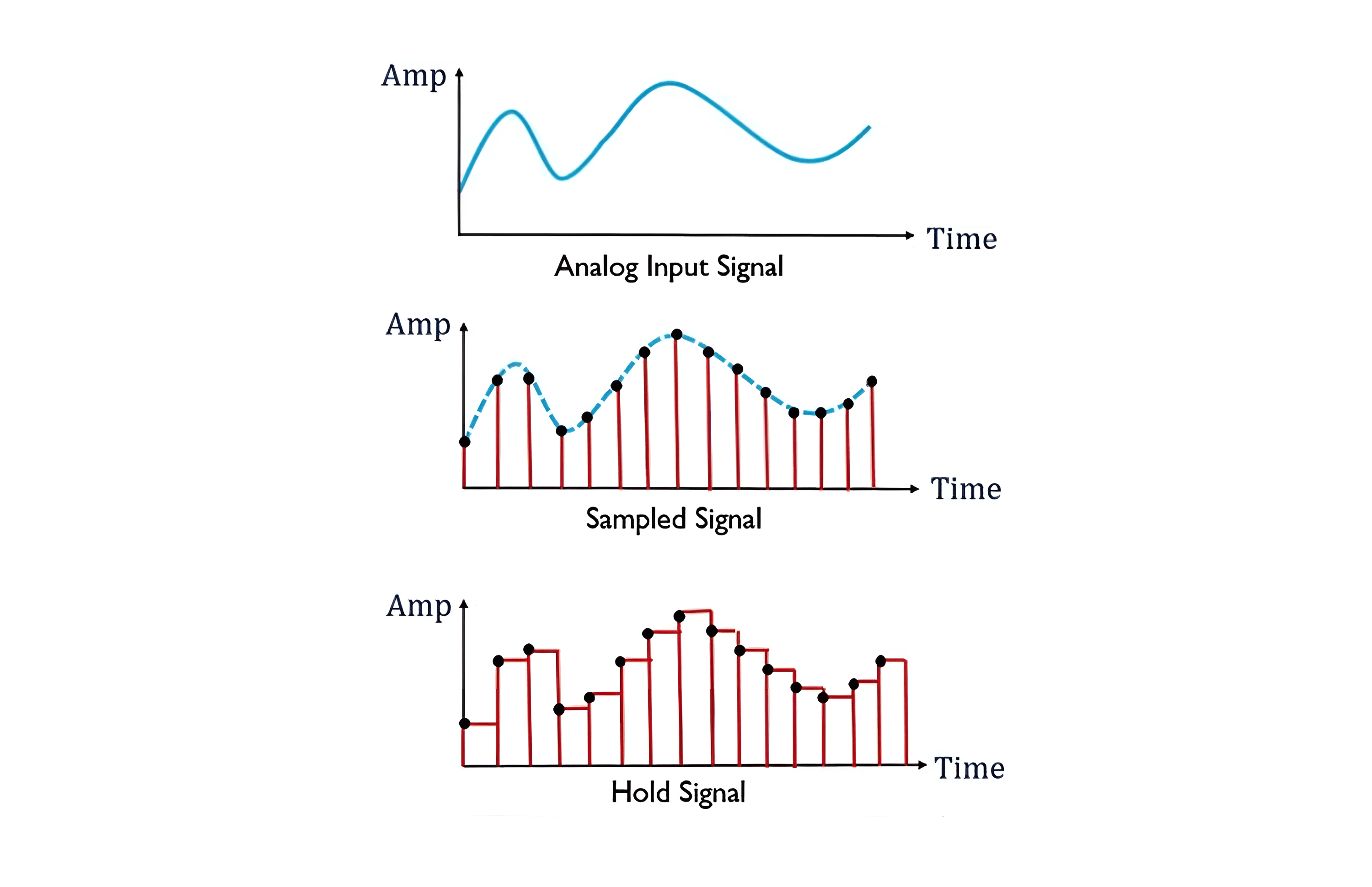### 保持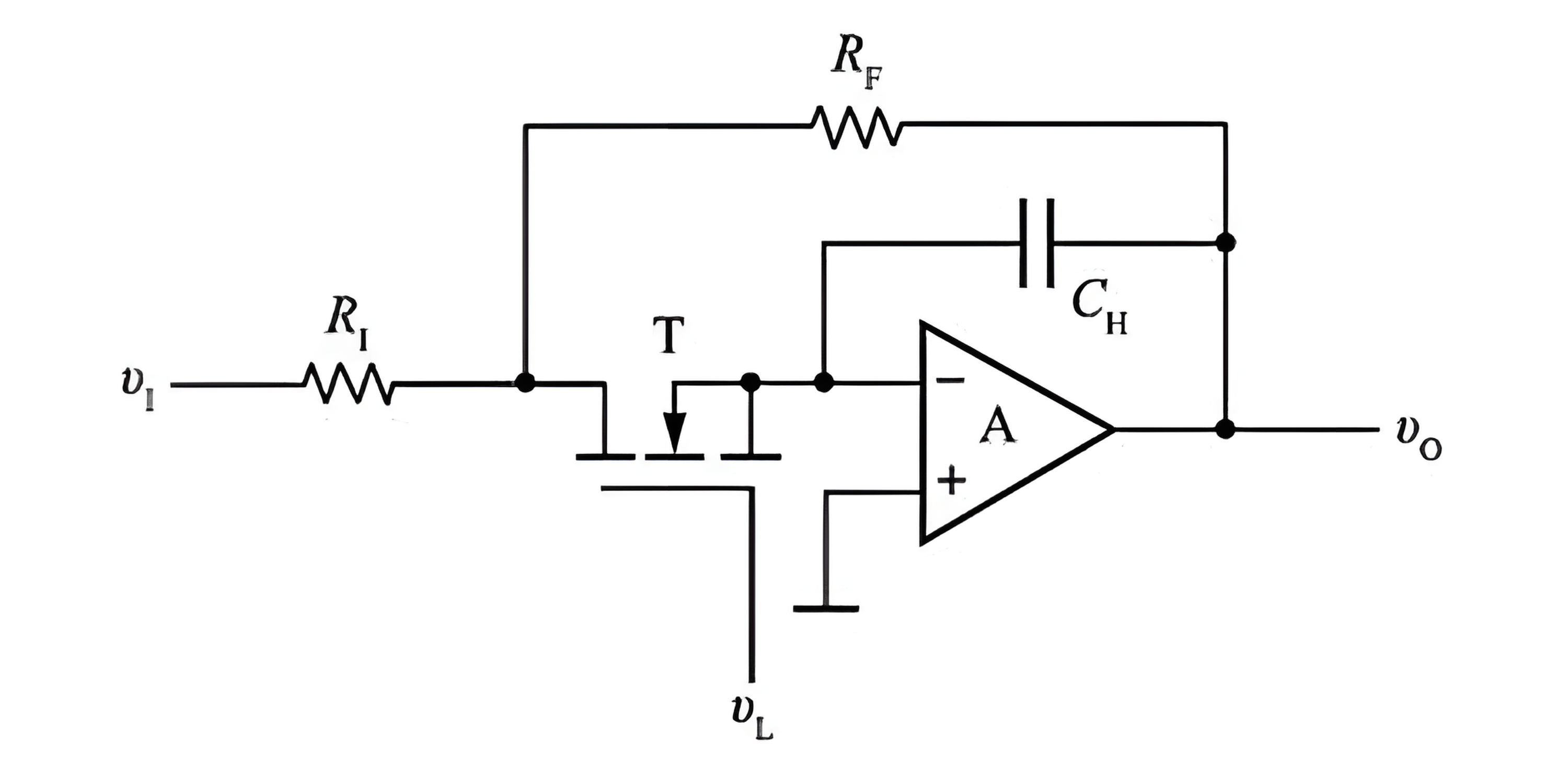1. 当采样控制信号 $$v_L$$ 为高电平时，使 MOS 管 $$T$$ 导通，$$v_1$$ 经过电阻 $$R_1$$ 和 MOS 管 $$T$$，给电容 $$C_H$$ 充电。
2. 若取 $$R_1=R_F$$，则充电结束后 $$v_0=v_c=-v_1$$
3. 当采样控制信号 $$v_L$$ 跌落回电平时，MOS 管 $$T$$ 截止，电容 $$C_H$$ 上的电压不会突变，所以 $$v_0$$ 也能保持一段时间，采样结果得以被记录下来。

### 并联比较型（Flash）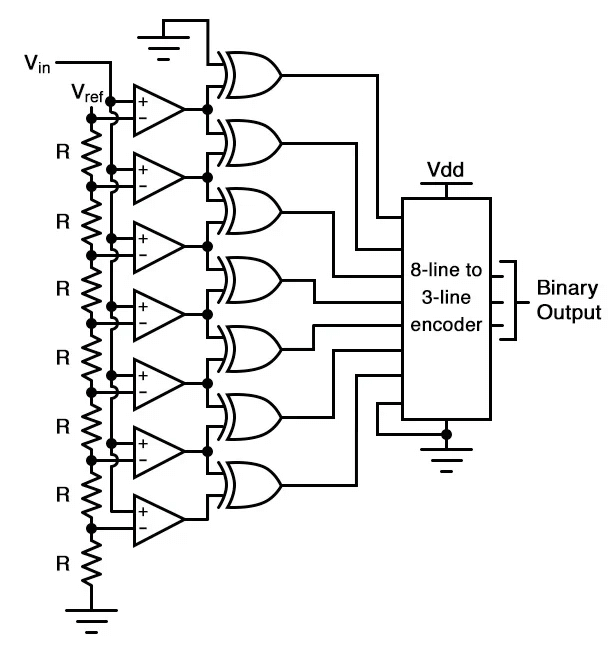### 逐次逼近型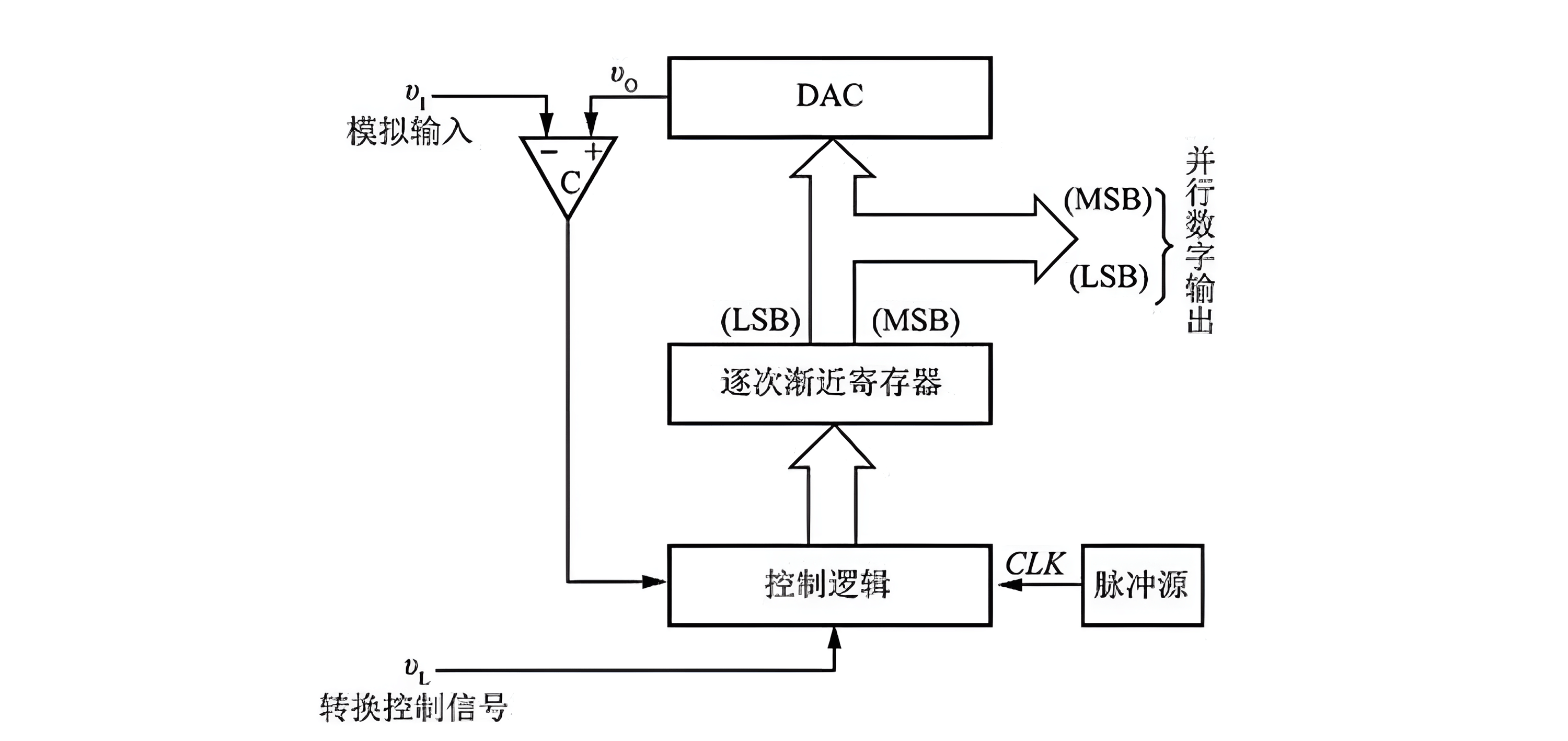### 双积分型（V-T）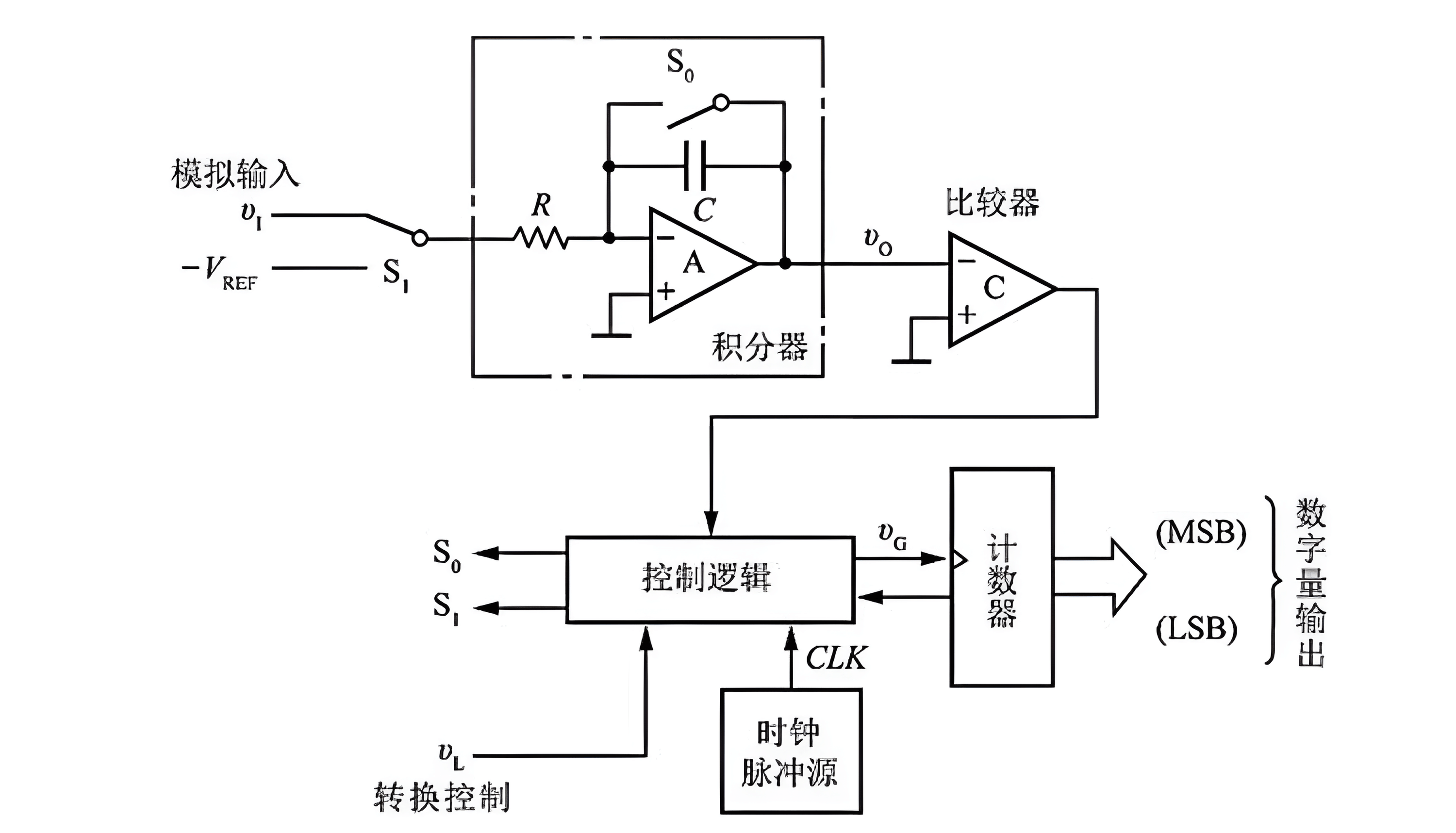### Σ-Δ 型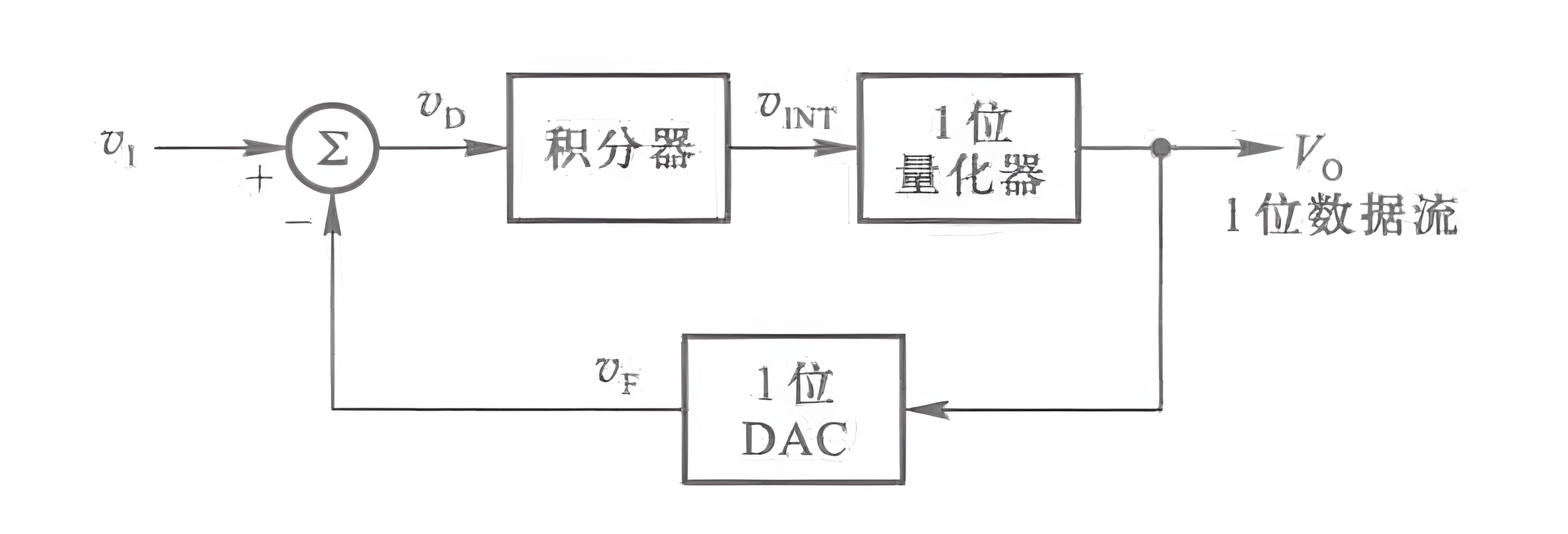### 电压 - 频率变换型（V-F）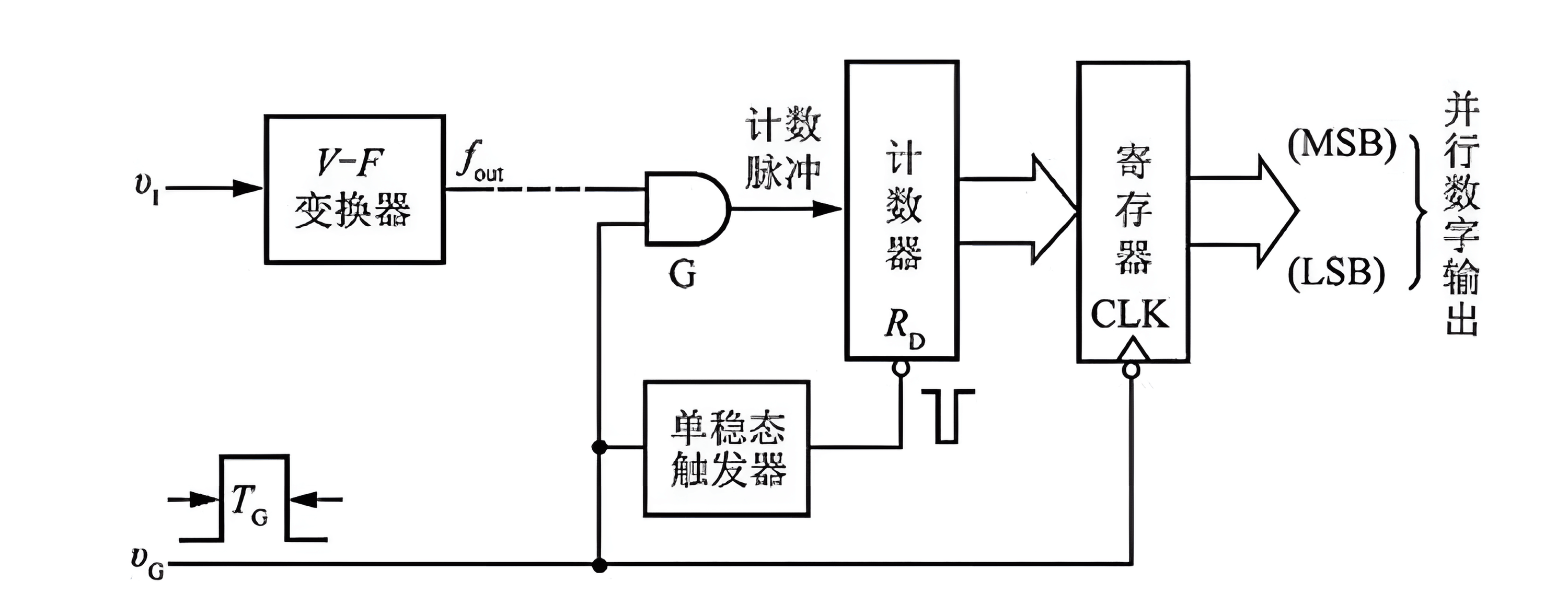• 将输入的模拟电压信号转换为对应的频率信号。
• 在固定的时间内对频信号率计数。
• 计数结果正比于输入电压的幅值。

• 分辨率：输出数字量变化一个相邻数值所需输入模拟电压的变化量，一般用二进制的位数表示，分辨率为 n 表示是满刻度 Fs 的 2 的 n 次方分之一。
• 转换速率：每秒进行转换的次数。

## DAC 基本原理

DAC（Digital-to-Analog Canverter），指数字 / 模拟转换器。可将数字量转换为成比例的模拟电压或电流。举个例子，计算机可能产生范围从 0000000011111111 的数字输出，DAC 将其转换为范围从 0 到 10V 的电压。DAC 从基本原理上可以分两类：电流求和型、分压器型。

## DAC 常见类型

### 开关树型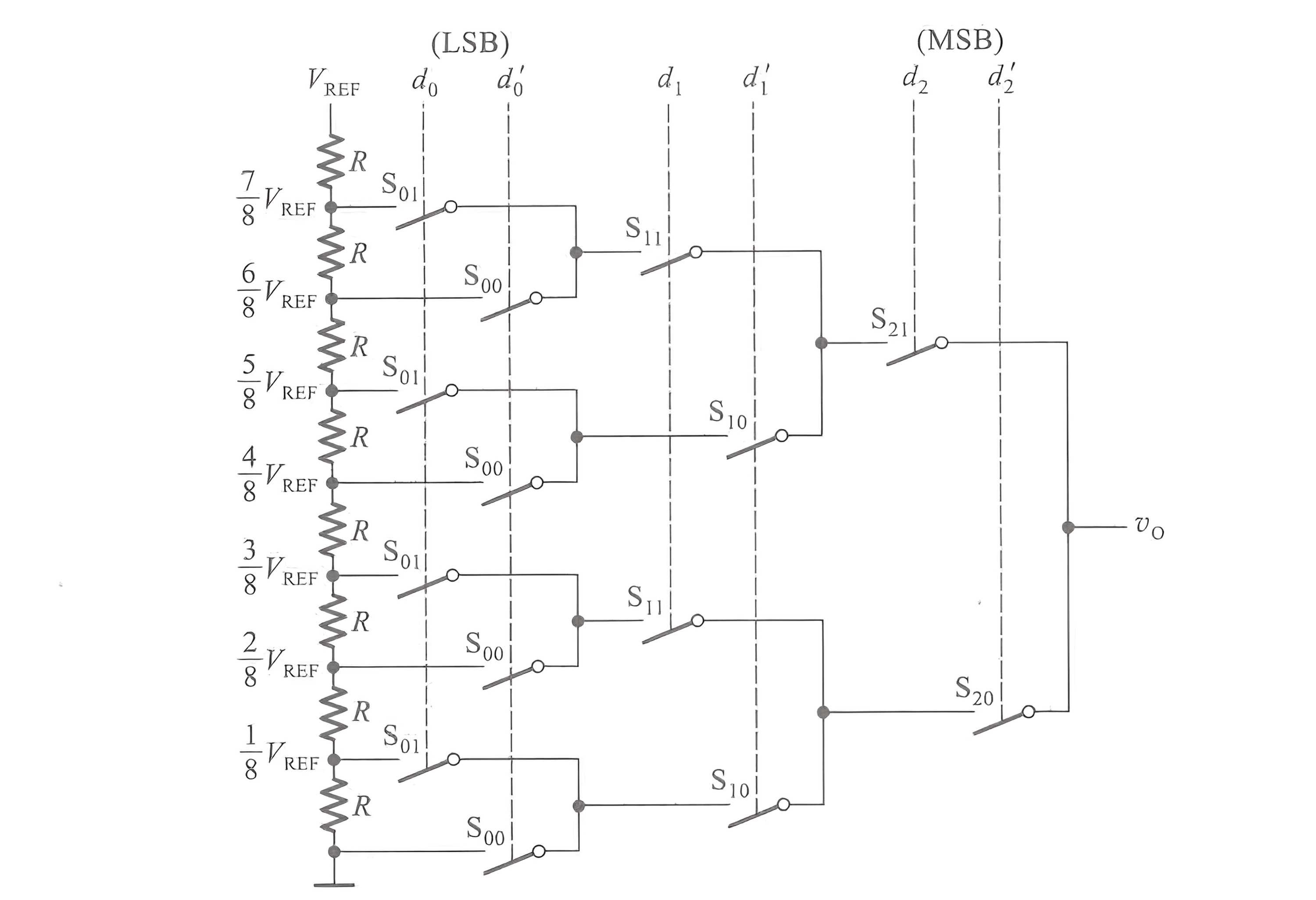$v_0=\frac{V_{REF}}{2^1} d_2+\frac{V_{REF}}{2^2} d_1+\frac{V_{REF}}{2^3} d_0$
$v_0=\frac{V_{REF}}{2^3} (d_2 2^2+d_1 2^1+d_0 2^0)$

$v_0=\frac{V_{REF}}{2^n} (d_{n-1} 2^{n-1}+d_{n-2} 2^{n-2}+...+d_1 2^1+d_0 2^0)$

### 权电阻网络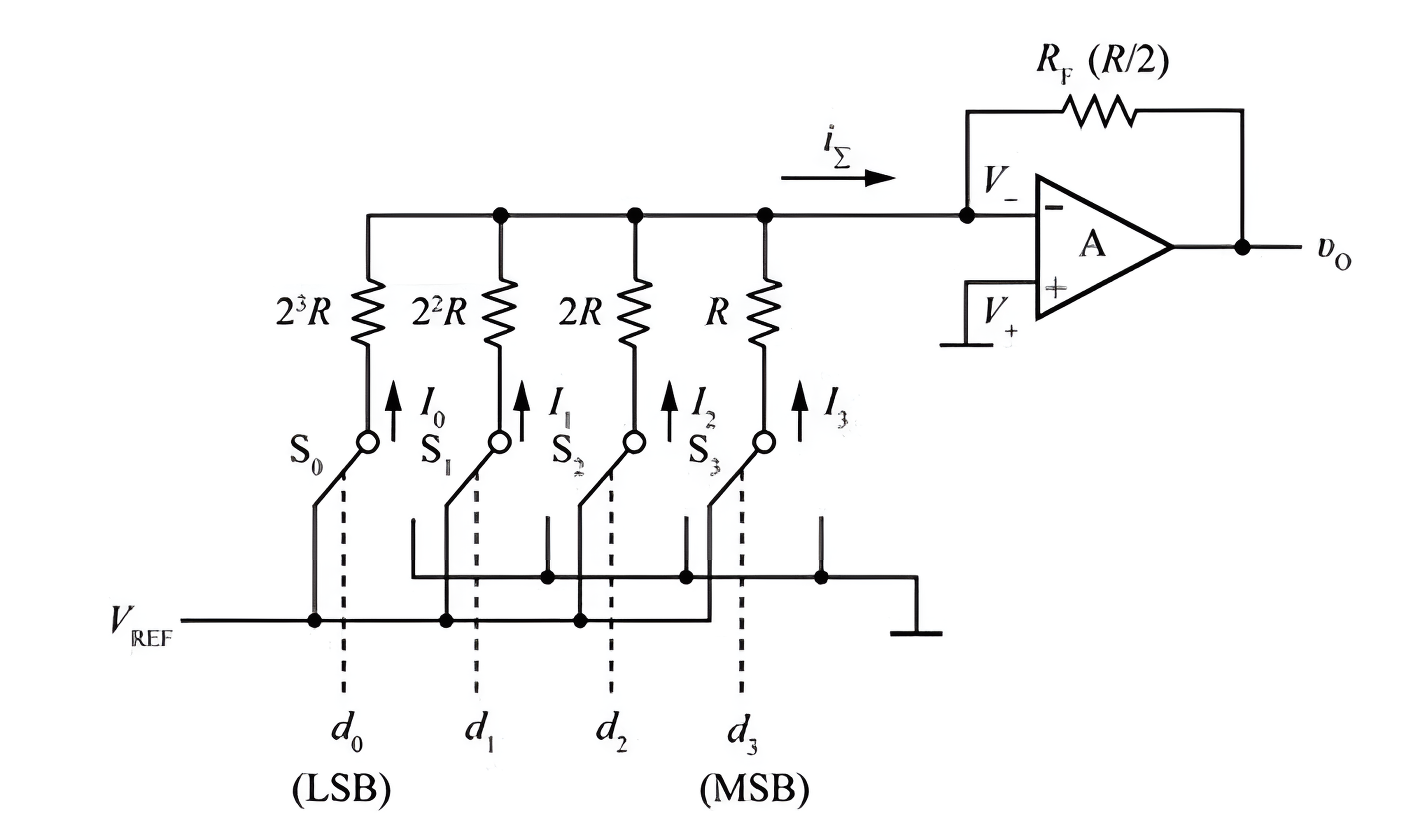$v_O=-R_F i_{\sum}=-R_F (I_3+I_2+I_1+I_0)$

$I_3=\frac{V_{REF}}{2^0 R} d_3$
$I_2=\frac{V_{REF}}{2^1 R} d_2$
$I_1=\frac{V_{REF}}{2^2 R} d_1$
$I_0=\frac{V_{REF}}{2^3 R} d_0$

$v_O=-\frac{V_{REF}}{2^4}(d_3 2^3+d_2 2^2+d_1 2^1+d_0 2^0)$

$v_O=-\frac{V_{REF}}{2^n}(d_{n-1} 2^{n-1}+d_{n-2} 2^{n-1}+...+d_{1} 2^{1}+d_{0} 2^{0})$
$v_O=-\frac{V_{REF}}{2^n}D_n$

### 倒 T 形电阻网络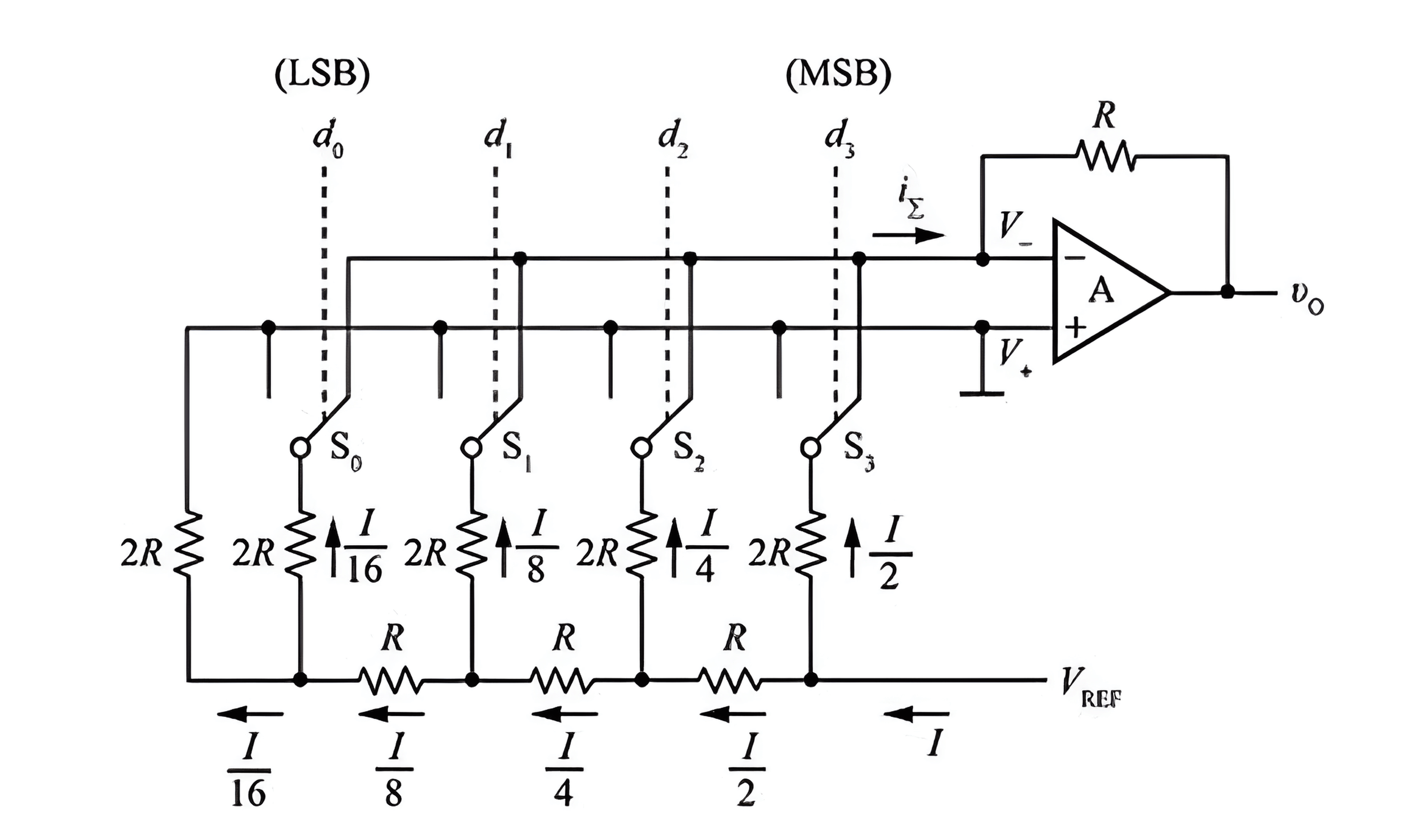$v_O=-Ri_{\sum}=-\frac{V_{REF}}{2^n}D_n$

### 权电流型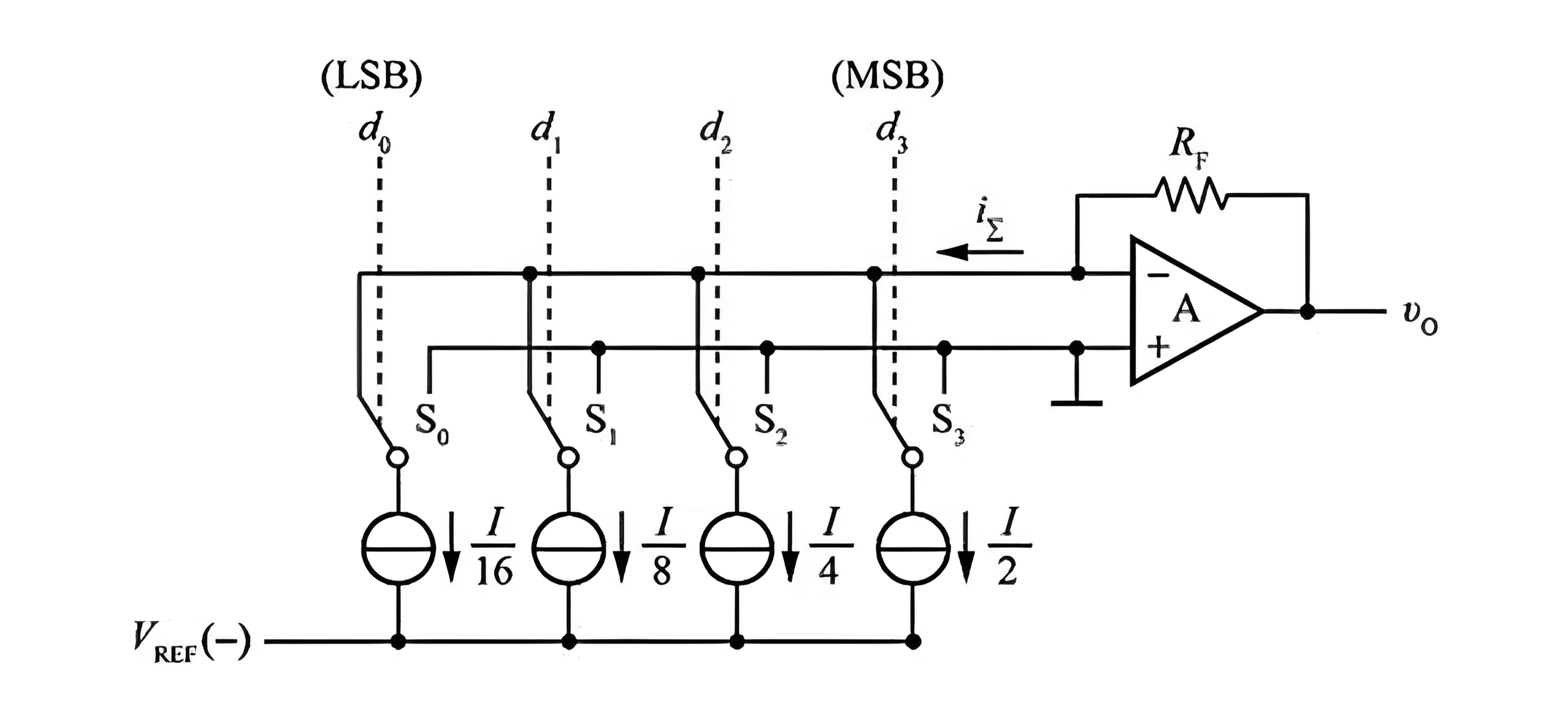$v_O=\frac{R_F V_{REF}}{2^n R_R}D_n$

## DAC 主要参数

• 分辨率：最小输出电压（也就是输入数字量为 1 时的电压）与最大输出电压（也就是输入数字量为最大，每一位都是 1 时的电压）之比。一般通过输入数字量的位数来表示。
• 转换量程：DAC 能输出的最大电压，一般的关于参考电压或其倍数。
• 建立时间：从输入数字量到输出模拟量之间的延时时间。